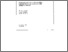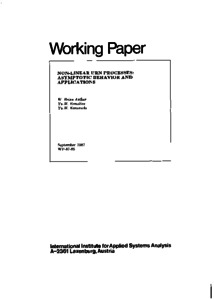Non-Linear Urn Processes: Asymptotic Behavior and Applications

Arthur, W.B., Ermoliev, Y.M., & Kaniovski, Y.M. (1987). Non-Linear Urn Processes: Asymptotic Behavior and Applications. IIASA Working Paper. IIASA, Laxenburg, Austria: WP-87-085Preview
Text
WP-87-085.pdf

Abstract

Adaptive (path dependent) processes of growth modeled by urn schemes are important for several fields of applications: biology, physics, chemistry, economics.

In this paper the authors continue their previous investigations of generalized urn schemes with balls of different colors and path-dependent increments. They consider processes with random additions of balls at each time. These processes are similar to the branching processes, however the classical theory of branching processes does not consider the case when the composition of added balls depends on the state of the process. These state dependent processes are very important for the applications and are considered in this paper.

The asymptotic behavior of the proportions of balls of each color in the total population is studied. It appears that these proportions can be expressed through the so-called urn functions which define the probabilities of adding the new ball depending on the current composition of urn population. The dynamics of the urn scheme is written in terms of a stochastic finite differential equation. The trajectories defined by this equation are attracted to the urn functions fixed points.

The techniques used to obtain these results have much in common with convergence analysis of the stochastic approximation type procedures for solving systems of nonlinear equations with discontinuous functions and stochastic quasi gradient procedures of stochastic optimization. In the general case the convergence results are formulated through nondifferentiable Lyapunov functions.

Item Type: Monograph (IIASA Working Paper) Adaption and Optimization (ADO)System and Decision Sciences - Core (SDS) IIASA Import 15 Jan 2016 01:57 27 Aug 2021 17:12 http://pure.iiasa.ac.at/2967View Item